Next: Astrophysical Relevance Up: Introduction Previous: Introduction   Contents

# The High-Temperature Phase Diagram

At the beginning, we will introduce the standard set of parameters used to describe plasmas and fluids. The degeneracy parameter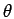is defined as the ratio of the temperature to Fermi temperature,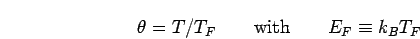(1)

where the Fermi temperature is taken from an ideal quantum gas of electrons in two spin states in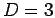dimensions,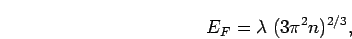(2)

where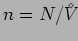is the density of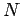atoms in the volume denoted by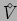. Fermionic effects become important at sufficiently low temperature when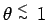. The density is often discussed in terms of the parameter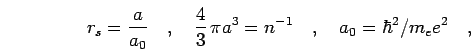(3)

where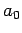denotes the electron Bohr radius and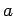the Wigner-Seitz radius. The coupling parameter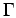describes the ratio of potential energy and kinetic energy,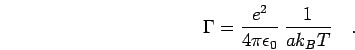(4)

Small values ofsuch as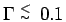describe a weakly coupled plasma, in which the Coulomb interaction can be treated as a correction to the dominating kinetic effects. In this limit, the Debye screening model in appendix C gives a reliable description.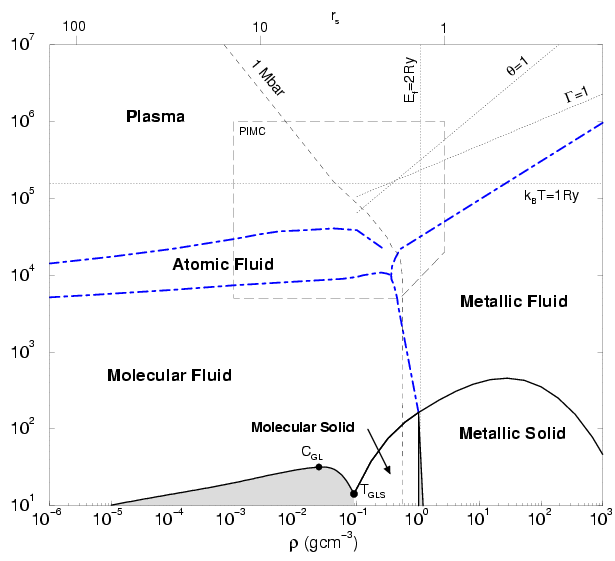The hydrogen phase diagram Fig. 1.1 was designed to show the various regimes of hydrogen and discuss the principle physical effects. It should be noted that several regions are not yet well understood e.g. how the high temperature results at 5000 K connect up to the room temperature regime. We used our estimates from PIMC simulations and extrapolated into different directions. In our approach, we do not consider relativistic effects, which become important when the thermal energy or the Fermi energy become of the order of the rest mass energy of the electron, which corresponds to a temperatures of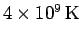and a density of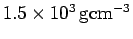.

In the low density and low temperature region of the phase diagram, hydrogen is composed of neutral species. Molecules dominate at low temperature (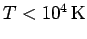), which dissociate into atoms with increasing temperature. If the temperature is increased further atoms become gradually ionized and a plasma of unbound electrons and protons is generated. Above the temperature corresponding to the binding energy of 1 Ry (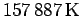) the probability for the occupation of bound states goes to zero.

Similarly in the limit of very high density, bound states cannot exist because the degeneracy effects dominate. There, delocalized states have a smaller energy than an antisymmetric combination of bound orbitals. This regime is expect to prevail when the Fermi energies become of the order 1 Ry. However, it should noted this represents a extremely simplified calculation and that the precise value probably lies significantly over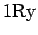. In the high-density limit, the electrons also form a rigid background and hydrogen behaves like a one-component plasma of ions with neutralizing background. In the phase diagram, we label this state as metallic fluid because the electrons behave like a degenerate Fermi gas and the estimated conductivity is high. The degeneracy also distinguishes this regime from the plasma state. We observed a continuous transition between the two regimes.

In the phase diagram, the region of PIMC simulations from this work combined with earlier ones by Pierleoni et al. (1994) and Magro et al. (1996) has been indicated. The region is of particular interest because there hydrogen is characterized by strong coupling, a substantial degree of degeneracy, but also by the formation of atom and molecules. It is very difficult to design a chemical model (see section 1.4) that includes all of the mentioned effects in a reliable approximation. In particular the interaction of neutral species with charged particles has been proven to be rather complicated. The advantage of the PIMC technique is that it is a quantum-statistical method, which includes all the mentioned effects just by considering protons and electrons interacting via the Coulomb potential. The method is exact except for requiring a nodal surface in order to deal with the fermion sign problem. In this work, we will derive a variational density matrix (VDM) that allows us to replace free particle nodes by a density matrix that includes interactions and bound states. It was found that the type of nodes begin to have a noticeable effect on the derived thermodynamic quantities for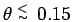.

Furthermore, there are also some practical limitations that put a limit on the applicability of the PIMC method in its current implementation, which originate from the available computational resources. For example, it has been proven to be difficult to go below temperatures of 5000 K because this would require paths with more 200 time slices in order to describe the formation of molecules accurately. Also the fermion nodes can reduce the efficiency for very high values of the degeneracy. Currently we are able to study hydrogen for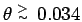. For very low density, the MC efficiency is reduced because particles rarely collide. This is also the case for PIMC but this limit has not been reached for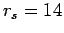. The reason why we have not performed simulations at higher temperature or lower density is that analytical models are expected to work very well in the regime of weak coupling.

The phase diagram also shows a region where the four discussed regimes: molecular, atomic, metallic fluid and plasma meet approximately. The region continues to be controversial. Many models have predicted a first-order plasma phase transition (PPT) with critical point and coexistence region of two fluids characterized by different degrees of ionization and densities. This existence of a PPT was first mentioned in a phase diagram by Landau and Zeldovich (1943). First calculations have been made by Norman and Starostin (1968) and Ebeling and Sändig (1973). Since then the research community has been divided. A number of different free energy models such as those by (Saumon and Chabrier, 1992; Kitamura and Ichimaru, 1998; Beule et al., 1999) predict a PPT. The exact location of the critical point and the coexistence region differ considerably. Other models show continuous transitions (Ross, 1998).

Also in the PIMC work by Magro et al. (1996), a first order phase transition between the molecular to a metallic phase was predicted. These predictions will be critically reviewed in this work. It will be discussed what effect the free particle nodes and the time step have on the nature of the transition.

The gas-liquid coexistence regime with the critical point and gas-liquid-solid triple point were taken from the work by Kitamura and Ichimaru (1998). The first order phase transition from molecular to metallic hydrogen was calculated by Ceperley and Alder (1987). From the work by Ceperley and Alder (1980) it is known that the Wigner crystal of electrons melts at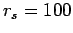. Similarly any solid structure of protons must become unstable if the proton parameter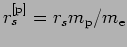reaches 100. That is the reason why the melting line of the metallic solid must decrease with temperature in the limit of high density.

Furthermore, it should noted that there exist different molecular phases in solid hydrogen at about 1 Mbar (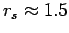), which have been studied intensely using diamond anvil cell experiments (Silvera and Pravica, 1998; Mao and Hemley, 1994) as well as with various simulation methods (Surh et al., 1997; Cui et al., 1997; Kitamura et al., 2000). The phases are labelled I, II and III and correspond to different orderings of the molecules in the crystal. Phase I is characterized by free rotation of the molecules represented by an angular momentum state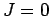. In Phase III, the rotational degree of freedom are frozen in and one refers to it as a state of classical orientational ordering. Phase II is believed to be distinguished by the ordering angular momentum states.Next: Astrophysical Relevance Up: Introduction Previous: Introduction   Contents
Burkhard Militzer 2003-01-15